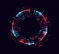## Using a single DSP48E2 Slice to infer three 48-bit inputs adderStarted by 4 years ago1 replylatest reply 7 months ago176 views

Hi All,
I have nine 8-bit values that I want to add using the dsp slices.

As an example I tried this code from the Xilinx answer records(https://www.xilinx.com/support/answers/66429.html).
I want to implement the second approach: "Two of the inputs are free to come from any source and one input comes from an internal DSP48 feedback signal as in a MAC."
But after synthesis even the original verilog code is inferring 2 DSP slices.
Am I interpreting things wrong or is there any bug in the code?

I created this VHDL equivalent of the Verilog code from Xilinx.

library IEEE;
use IEEE.STD_LOGIC_1164.ALL;
use IEEE.NUMERIC_STD.ALL;

GENERIC(
CONSTANT SIZEIN  : NATURAL := 48; 	-- Input size
CONSTANT SIZEOUT : NATURAL := 48 	-- Output size
);
PORT(
clk          : in STD_LOGIC;	-- Clock
resetn_glb   : in STD_LOGIC; 	-- Global Reset from the Zynq PS
resetn_lc    : in STD_LOGIC; 	-- Local Reset if necessary
operand_i1   : in  STD_LOGIC_VECTOR (SIZEIN-1 downto 0);	-- 1st input to dsp
operand_i2   : in  STD_LOGIC_VECTOR (SIZEIN-1 downto 0);	-- 2nd input to dsp
operand_i3   : in  STD_LOGIC_VECTOR (SIZEIN-1 downto 0);	-- 3nd input to dsp
avg_out      : out STD_LOGIC_VECTOR (SIZEOUT-1 downto 0)	-- Averged output
);
attribute USE_DSP : string;
attribute USE_DSP of tri_adr: entity is "YES";
end ENTITY;

signal a    : std_logic_vector (26 downto 0);
signal b    : std_logic_vector (17 downto 0);
signal pcout    : std_logic_vector (SIZEOUT-1 downto 0);
signal avg_t    : std_logic_vector (SIZEOUT-1 downto 0);

begin
avg:PROCESS (clk)		--check every clk
begin
if rising_edge(clk) then
if resetn_glb = '0' then	--negative assert
avg_t <= (OTHERS => '0');
pcout <= (OTHERS => '0');
else

pcout  <= std_logic_vector((signed(a) * signed(b)) + signed(operand_i1));
avg_t  <= std_logic_vector(signed(pcout) + signed(operand_i2) + signed(operand_i3));
--				avg_t  <= std_logic_vector(signed(operand_i1) + signed(operand_i2) + signed(operand_i3));
end if;
end if;
end PROCESS;

-- Output result
avg_out <= avg_t;

end behav;

I will be adding three 8-bit numbers using one DSP slice and create a cascaded adder logic to do addittion of all nine values.